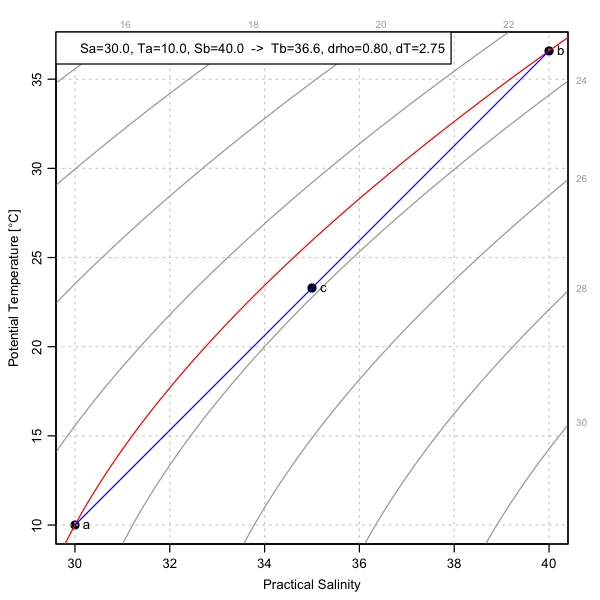Abstract R code is provided in aide of laboratory demonstration of cabelling.

# Introduction

Setting up a cabelling experiment requires creating two watermasses of equal density, and if only S and T can be measured, that means calculating densities. Using a TS diagram and graphical interpolation is one approach to that task, but another is to use R to do the calculation.

# Methods

The code given below will do the calculation for specified `Sa`, `Ta` and `Sb`, where the second letter indicates the watermass. The code uses `uniroot()` to find the temperature `Tb` that yields equal densities for watermasses a and b.

If run non-interactively, the code will produce a PNG file like that given below.

# Results

The legend summarizes the results, indicating also the density change and the temperature change that would be equivalent to that density change (at the midpoint, c).# Conclusions

If the design goal is that the density mismatch between watermasses a and b should be, say, 10 percent of the density difference of the mixture watermass (c), then in the illustrated case the temperature would have to be controlled to within a quarter of a degree Celcius – a task that is challenging enough to argue against this as an informal classroom demonstration.

# Exercises

1. Alter the R code to calculate `Sb` in terms of `Tb`.
2. Consider (calculate or measure) the convection associated with sidewall heat conduction.

This website is written in Jekyll, and the source is available on GitHub.